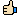The difference between sequential patterns and sequential rules
Date: May 28, 2012 04:28AM

Hello,

Today I received the following question by e-mail.

What is the difference between sequential pattern and sequential rules?

I will share my answer with everyone.

The difference is simple. Consider the following sequence database containing four sequences:

s1: {a}, {b}, {c}, {e}
s1: {a}, {b}, {c}, {e}
s1: {a, f}, {b, g}, {c, h},
s1: {a}, {b}, {g}, {h}

A sequential pattern is described by its support.

For example, {a},{b}, {c}, {e} is a sequential pattern. It has a support of 50% because it appears in two out of four sequences from the sequence database.

However, for many applications knowing the support is often not enough. If we only consider the support, the patterns may be misleading For example, consider that the previous database represents customer buying behavior. If a customer buys {a}, {b}, would you recommend to buy {c}, {e} based on the pattern {a},{b}, {c}, {e} ? It may not be a good idea because if you look carefully, {a},{b} appears in four sequences. But it is only followed by {c},{e} in half of the sequences.

To have a better idea of the probability that a pattern will be followed, we can add the concept of confidence.

Sequential rules are based on this idea. A sequential rule has a support. But it also has a confidence. This is the main difference with sequential patterns.

For example, the sequential rule {a,b} --> {c,e} has a support of 50% and a confidence of 50% because a,b is followed by c,e only in 50 % of the cases.

The confidence is simply calculated as the number of sequences that contain the rule divided by the number of sequences that contain its antecedent (left part).

There are different kind of sequential rules. Here, I have described the kind that I use in my work and that is available with the TopSeqRules, RuleGrowth, TRuleGrowth, CMRules and CMDeo algorithm in SPMF.

The papers describing these algorithms can be found on my website:

http://www.philippe-fournier-viger.com/publications.php

There is also some algorithms that exist for mining sequential rule in a single sequence instead of a sequence database. These rules are usually called "episode rules".

Philippe

Re: The difference between sequential patterns and sequential rules
Posted by: Dvijesh88
Date: May 31, 2012 09:22PMreally good describe sir